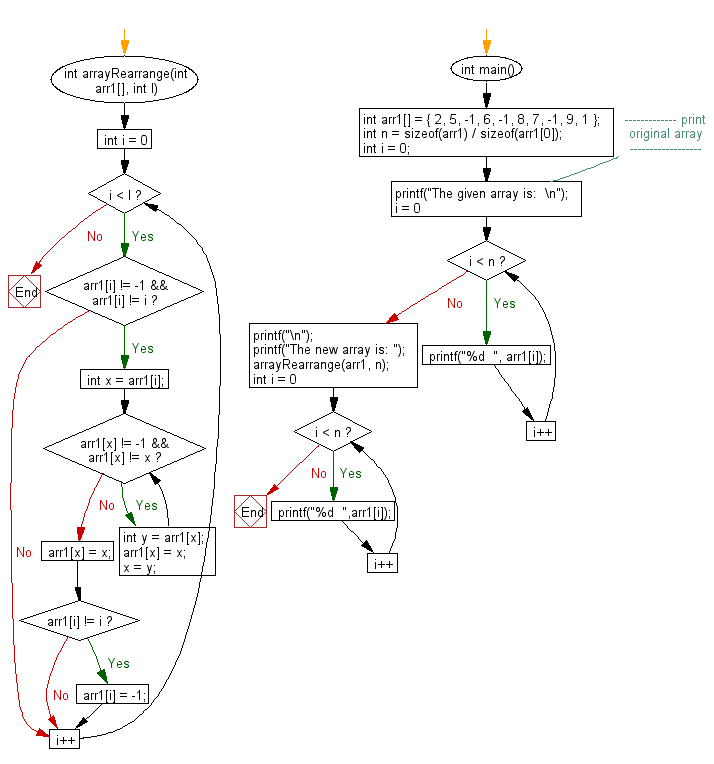﻿ C exercises: Rearrange an array such that arr[i]=i - w3resource# C Exercises: Rearrange an array such that arr[i]=i

## C Array: Exercise-101 with Solution

Write a program in C to rearrange an array such that arr[i]=i.

N.B.: Given array contains N elements, from 0 to N – 1. All elements within the range may not be present in the array. There will be -1 if an element within the range is not present in the array.

Sample Solution:

C Code:

``````#include<stdio.h>

int arrayRearrange(int arr1[], int l)
{
for (int i = 0; i < l; i++)
{
if (arr1[i] != -1 && arr1[i] != i)
{
int x = arr1[i];
while (arr1[x] != -1 && arr1[x] != x)
{
int y = arr1[x];
arr1[x] = x;
x = y;
}
arr1[x] = x;
if (arr1[i] != i)
{
arr1[i] = -1;
}
}
}
}

int main()
{
int arr1[] = { 2, 5, -1, 6, -1, 8, 7, -1, 9, 1 };
int n = sizeof(arr1) / sizeof(arr1);
int i = 0;
//------------- print original array ------------------
printf("The given array is:  \n");
for(i = 0; i < n; i++)
{
printf("%d  ", arr1[i]);
}
printf("\n");
//-----------------------------------------------------------

printf("The new array is: ");
arrayRearrange(arr1, n);
for (int i = 0; i < n; i++)
printf("%d  ",arr1[i]);
}
```
```

Sample Output:

```The given array is:
2  5  -1  6  -1  8  7  -1  9  1
The new array is: -1  1  2  -1  -1  5  6  7  8  9
```

Pictorial Presentation:Flowchart:C Programming Code Editor:

Improve this sample solution and post your code through Disqus.

﻿